Question

# (Hardy Weinberg Equation) In a population of rabbits, there are 423 A1A1, 1484 A2A2, and 1661...

(Hardy Weinberg Equation)

In a population of rabbits, there are 423 A1A1, 1484 A2A2, and 1661 A1A2 individuals. If the enviornment changes so that the homozygous recessive genotype suffers a reduction of fitness where it's fitness is now 0.59 , but the other genotypes are unaffected, what will be the frequency of the dominant allele in the NEXT generation?

Note: Assuming A1 dominant to A2.

Given, relative fitness of A1A1 = A1A2 = 1, relative fitness of A2A2 = 0.59, number of A1A1 = 423, number of A2A2 = 1484, number of A1A2 = 1661

So, total number of rabbits = 423 + 1484 + 1661 = 3568

So, frequency of A1A1 = Number of A1A1 / Total population = 423/3568 = 0.11855 (Up to 5 decimals)

So, frequency of A1A2 = Number of A1A2 / Total population = 1661/3568 = 0.46553 (Up to 5 decimals)

So, frequency of A2A2 = Number of A2A2 / Total population = 1484/3568 = 0.41592 (Up to 5 decimals)

Now, mean fitness = (Frequency of A1A1 x Fitness of A1A1) + (Frequency of A1A2 x Fitness of A1A2) + (Frequency of A2A2 x Fitness of A2A2) = (0.11855 x 1) + (0.46553 x 1) + (0.41592 x 0.59) = 0.11855 + 0.46553 + 0.24539 = 0.82947

So, after selection frequency of A1A1 = (Frequency of A1A1 x Fitness of A1A1) / mean fitness = 0.11855 / 0.82947 = 0.14292 (Up to 5 decimals)

So, after selection frequency of A1A2 = (Frequency of A1A2 x Fitness of A1A2) / mean fitness = 0.46553 / 0.82947 = 0.56124 (Up to 5 decimals)

So, frequency of A1 (Dominant allele) in the next generation = 1/2 of frequency of A1A2 + Frequency of A1A1 = 1/2 x 0.56124 + 0.14292 = 0.42354

#### Earn Coins

Coins can be redeemed for fabulous gifts.

Similar Homework Help Questions
• ### The Hardy-Weinberg principle and its equations predict that frequencies of alleles and genotypes remain constant from generation to generation in populations that are not evolving

.1. The Hardy-Weinberg principle and its equations predict that frequencies of alleles and genotypes remain constant from generation to generation in populations that are not evolving. What five conditions does this prediction assume to be true about such a population? a._______  b._______  c._______  d._______  e._______  2. Before beginning the activity, answer the following general Hardy-Weinberg problems for practice (assume that the population is at Hardy-Weinberg equilibrium).a. If the frequency of a recessive allele is 0.3, what is the frequency of the dominant...

• ### In a population of mice a particular locus has two alleles A1 (dominant) and A2 (recessive)....

In a population of mice a particular locus has two alleles A1 (dominant) and A2 (recessive). There are 126 A1A1, 167 A1A2 and 88 A2A2. Is this population in Hardy-Weinberg equilibrium (3 pts)? In a population of Gragons, there are 3151 A1A1, 1678 A1A2 and 2014 A2A2 individuals. If the environment changes so that the homozygous recessive genotype suffers a reduction of fitness where its fitness is now 0.73, but the other genotypes are unaffected, what will be the frequency...

• ### Scientists have identified three genotypes (A1A1, A1A2, A2A2) at a locus encoding the enzyme peroxidase in...

Scientists have identified three genotypes (A1A1, A1A2, A2A2) at a locus encoding the enzyme peroxidase in Ponderosa pine trees growing at Crater Lake. The observed number of individuals we these genotypes are given below: A1A1 = 135 A1A2 = 44 A2A2 = 11 Calculate the EXPECTED number of individuals with each genotype, as predicted under Hardy-Weinberg equilibrium. Carry out a chi-square goodness-of-fit test on the observed and expected numbers of individual Ponderosa pine trees given. What is the chi-square value...

• ### If the genotypes A1A1, A1A2, and A2A2 have frequencies 0.5, 0.25, and 0.25 respectively, what are...

If the genotypes A1A1, A1A2, and A2A2 have frequencies 0.5, 0.25, and 0.25 respectively, what are the frequencies of the A1 and A2 alleles? b) After a single generation of random mating, what are the expected frequency of A1A1, A1A2 and A2A2 in the offspring? c) If A2 is a recessive allele causing freckles, how many freckled offspring do you expect if there are 441 offspring in total?

• ### Evolution 1. Assume this population is in Hardy-Weinberg equilibrium. In a population of 120 cats, 35...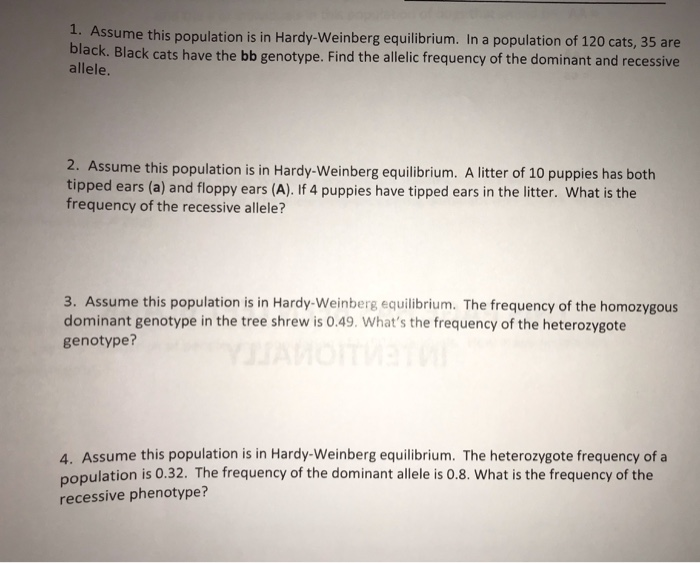Evolution 1. Assume this population is in Hardy-Weinberg equilibrium. In a population of 120 cats, 35 are black. Black cats have the bb genotype. Find the allelic frequency of the dominant and recessive allele. 2. Assume this population is in Hardy-Weinberg equilibrium. A litter of 10 puppies has both tipped ears (a) and floppy ears (A). If 4 puppies have tipped ears in the litter. What is the frequency of the recessive allele? 3. Assume this population is in Hardy-Weinberg...

• ### 2.3 Problem 3 The Hardy-Weinberg equation is useful for predicting the percent of a hu- man...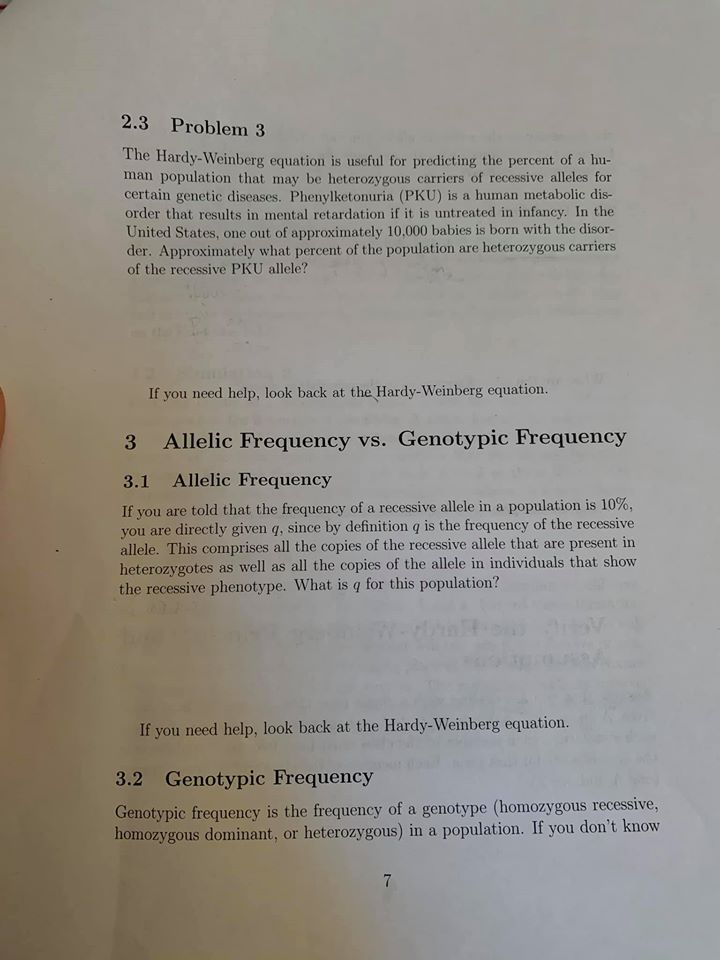2.3 Problem 3 The Hardy-Weinberg equation is useful for predicting the percent of a hu- man population that may be heterozygous carriers of recessive alleles for certain genetic diseases. Phenylketonuria (PKU) is a human metabolic dis- order that results in mental retardation if it is untreated in infancy. In the United States, one out of approximately 10.000 babies is born with the disor- der. Approximately what percent of the population are heterozygous carriers of the recessive PKU allele? If you...

• ### reting Data: Hardy-Weinberg Equation 2 of 10 you use the Hardy Weinberg equation to answer questions...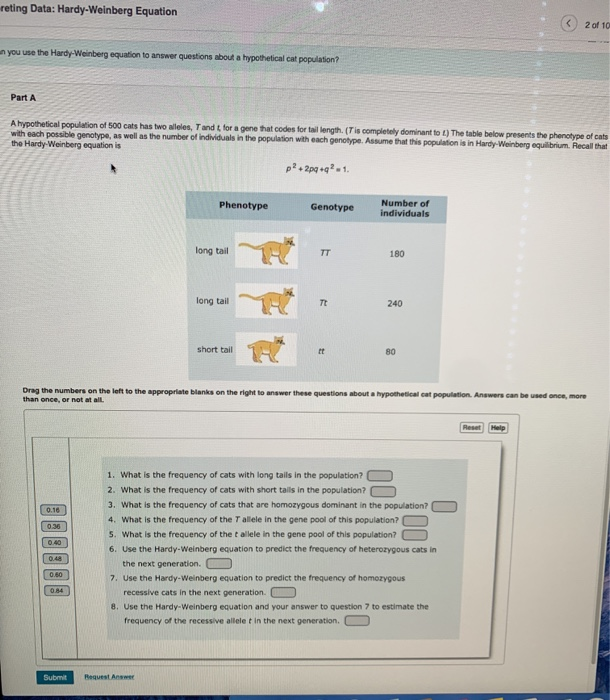reting Data: Hardy-Weinberg Equation 2 of 10 you use the Hardy Weinberg equation to answer questions about a hypotheticalcat population Part A A hypothetical population of 500 cats has two wees, Tandt for a gene that codes for tail length (Tis completely dominantot) The table below presents the phenotype of cats with each possible genotype, as well as the number of individuals in the population with each genotype. Assume that this population is in Hardy-Weinberg equilibrium Recall that the Hardy...

• ### At a biallelic locus, suppose that genotypes A1A1, A1A2, and A2A2 have relative fitnesses 1, 1.02,...

At a biallelic locus, suppose that genotypes A1A1, A1A2, and A2A2 have relative fitnesses 1, 1.02, and 1.03 and that the frequency of A1 is p = 0.1. (a) What is the population’s mean relative fitness? (b) What are the “marginal” or allele-specific relative fitnesses of A1 and A2? (c) What is the expected frequency of A1 in the following generation?

• ### 5. Examine the following fabricated data for a population of oak trees. Genotype Height Number of...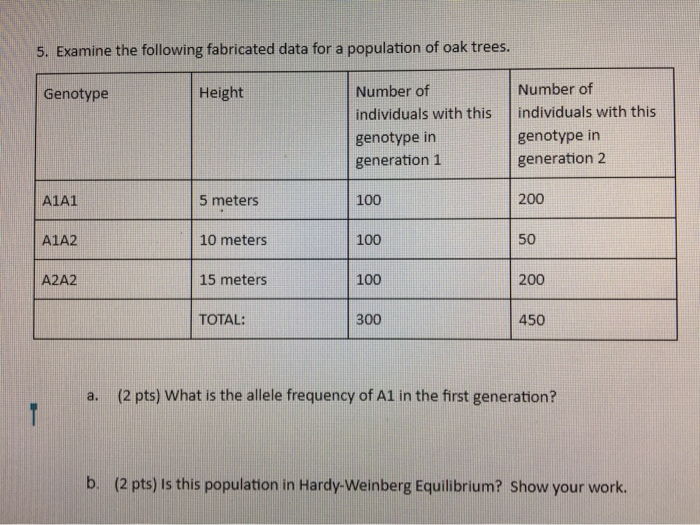5. Examine the following fabricated data for a population of oak trees. Genotype Height Number of individuals with this genotype in generation 1 Number of individuals with this genotype in generation 2 A1A1 5 meters 100 200 A1A2 10 meters 100 A2A2 15 meters 100 200 TOTAL: 300 450 a. (2 pts) What is the allele frequency of A1 in the first generation? b. (2 pts) is this population in Hardy-Weinberg Equilibrium? Show your work.

• ### Consider a population of 5694 adults, with the following numbers of different genotypes: A1A1 - 15...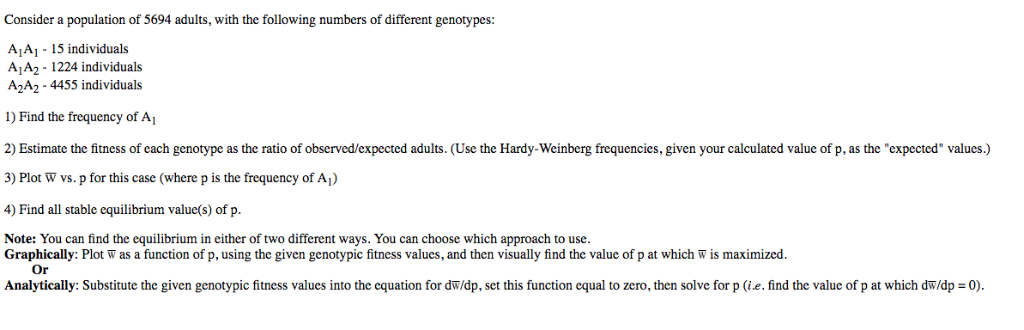Consider a population of 5694 adults, with the following numbers of different genotypes: A1A1 - 15 individuals A1A2- 1224 individuals A2A2 - 4455 individuals ) Find the frequency of A 2) Estimate the fitness of each genotype as the ratio of observed/expected adults. (Use the Hardy-Weinberg frequencies, given your calculated value of p, as the "expected" values.) 3) Plot W vs. p for this case (where p is the frequency of Aj) 4) Find all stable equilibrium value(s) of p....